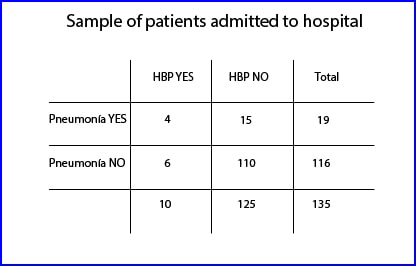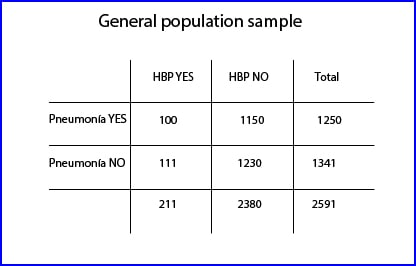# Generalizations are hazardous

Tabla de contenidos

# Berkson’s fallacy

We all like to generalize and statisticians and epidemiologists more than anyone. After all, one of the main purposes of these two sciences is to apply conclusions to an inaccessible population drawn from the results get in a smaller and therefore more manageable sample.

For example, when we perform a study about the effect of a risk factor for a particular disease, we usually do it with a small number of cases, which is our sample, but to draw conclusions that we can extrapolate to the whole population. Of course, to do so, we need the sample is appropriate and representative of the population we want to generalize the results. Let’s see an example of what happens when this assumption is not met.Suppose we want to study whether the subjects affected of pneumonia are more likely to have high blood pressure. If we go to the easiest course we can use our database of hospital admissions and get from it our study sample as observed in the first table. We see that our sample includes 135 patients who required hospital admission, 19 of whom had pneumonia and four, in addition, hypertension. On the other hand, we can see that the number of hypertensive subjects is 10, four with pneumonia and six without it.

First, let’s see if there is any association between the two variables. So, we can make a chi-square’s test under the null hypothesis of no association. I used the R program to calculate it. First I built the table with the following command:

and then I calculated the chi-squared test applying the Yates correction (there is a cell with lower than five instances):

and I get a value of chi = 3.91, that for one degree of freedom corresponds to a value of p = 0.04. As it is less than 0.05, I reject the null hypothesis of no association and concluded that there is an association between the two variables.

Now, to calculate the strength of the association I calculate the odds ratio, using any of the epidemiology calculators available on the Internet. The odds ratio is 4.89, with a 95% confidence interval from 1.24 to 19.34, so we concluded that patients with pneumonia are nearly five times more likely to have hypertension.

## Berkson’s fallacy

And so far everything is going well. The problem would arise if we succumb to the temptation to generalize the results to the general population. And this is because the odds ratio measures the strength of association between two variables provided that the sample was obtained randomly, which is not our case. Let’s see what happens if we repeat the experiment with a larger sample obtained, not from our hospital record, but from the general population (that includes the participants of the first experiment).We thus obtain the second contingency table, which includes 2591 patients, 211 of whom are hypertensive. Following the same procedure of the first experiment, first we calculate the chi-square, which in this case has a value of 1.86, which corresponds to a p = 0.17. Being more than 0.05 we cannot reject the null hypothesis, so we must conclude that there is no association between the two variables.

It no longer makes much sense to calculate the odds ratio, but we can do it and see that it values 0.96, with a 95% confidence interval from 0.73 to 1.21. Because the interval includes the value of one, the odds ratio is not significant.

Why does it occur this difference between the two results? This is because the risk of hospitalization is different among different groups. Of the 100 individuals who have pneumonia (second table), four require admission (first table), then the risk is 4/10 = 0.4. The risk among those with hypertension alone is 6/111 = 0.05, and of those who have no disease is 110/1230 = 0.09.

Thus, we see that patients with pneumonia are more likely than the rest of being hospitalized. If we make the mistake of including only hospitalized patients, our results will be biased relative to the general population, thus observing an association that actually does not exist. This type of spurious association between variables that is caused by an incorrect choice of the sample is known as the Berkson’s fallacy.

## We’re leaving…

And here we end for today. We see that how we choose the sample is of paramount importance when generalizing the results of a study. This is what usually happens with clinical trials with strict inclusion criteria, that it is difficult to generalize the results. So there are authors who prefer pragmatic clinical trials, more stuck to everyday reality and more generalizable. But that’s another story…

This site uses Akismet to reduce spam. Learn how your comment data is processed.

Esta web utiliza cookies propias y de terceros para su correcto funcionamiento y para fines analíticos. Al hacer clic en el botón Aceptar, aceptas el uso de estas tecnologías y el procesamiento de tus datos para estos propósitos. Antes de aceptar puedes ver Configurar cookies para realizar un consentimiento selectivo.    Más información